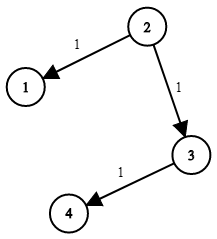# GeetCode Hub

You are given a network of `n` nodes, labeled from `1` to `n`. You are also given `times`, a list of travel times as directed edges `times[i] = (ui, vi, wi)`, where `ui` is the source node, `vi` is the target node, and `wi` is the time it takes for a signal to travel from source to target.

We will send a signal from a given node `k`. Return the time it takes for all the `n` nodes to receive the signal. If it is impossible for all the `n` nodes to receive the signal, return `-1`.

Example 1:```Input: times = [[2,1,1],[2,3,1],[3,4,1]], n = 4, k = 2
Output: 2
```

Example 2:

```Input: times = [[1,2,1]], n = 2, k = 1
Output: 1
```

Example 3:

```Input: times = [[1,2,1]], n = 2, k = 2
Output: -1
```

Constraints:

• `1 <= k <= n <= 100`
• `1 <= times.length <= 6000`
• `times[i].length == 3`
• `1 <= ui, vi <= n`
• `ui != vi`
• `0 <= wi <= 100`
• All the pairs `(ui, vi)` are unique. (i.e., no multiple edges.)

class Solution { public int networkDelayTime(int[][] times, int n, int k) { } }